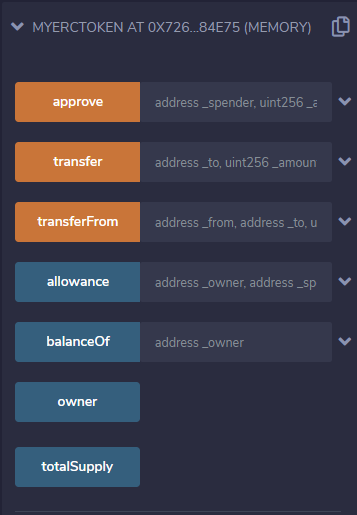# Solidity – Programming ERC-20 Token

ERC token standard 20 tokens in a smart contract token that follows the Ethereum Request for Comment proposal 20 guidelines. Our program will have the following mandatory functions:

• function totalSupply() public view returns (uint256);
• function balanceOf(address tokenOwner) public view returns (uint);
• function transfer(address to, uint tokens) public returns (bool);
• function approve(address spender, uint tokens)  public returns (bool);

And two events which emit to the external application:

Important keywords used:

• Event: An event is Solidity’s way of allowing clients e.g notifying your front end application about the occurrence of a specific event.
• View: View functions ensure that they will not modify the state
• public: A public function can be accessed outside the contract itself
• indexed: Up to three parameters can receive the attribute indexed through which we can search respective arguments.

Step 1: The expression mapping(address => uint256) defines an associative array whose keys are of type address and value of type uint256 used to store the balance.

1. balances will hold the token balance of each owner’s account.
2. allowed will include all the accounts approved to withdraw from a given account together with the withdrawal sum allowed for each.
```mapping(address => uint256) balances;

Step 2: Define the total supply of the token.

Step 3: Declare the events required and add the compulsory functions as per the specified signature.

## Solidity

 `// Compatible with version` `// of compiler upto 0.6.6` `pragma solidity ^0.6.6; `   `// Creating a Contract` `contract` `GFGToken` `{`   `// Table to map addresses ` `// to their balance` `mapping(address => uint256) balances;`   `// Mapping owner address to ` `// those who are allowed to ` `// use the contract` `mapping(address => mapping (` `        ``address => uint256)) allowed;`   `// totalSupply` `uint256 _totalSupply = 500; `   `// owner address` `address ``public` `owner; `   `// Triggered whenever ` `// approve(address _spender, uint256 _value) ` `// is called.` ` ``event Approval(address indexed _owner, ` `                ``address indexed _spender, ` `                ``uint256 _value);`   `// Event triggered when ` `// tokens are transferred.` `event Transfer(address indexed _from, ` `               ``address indexed _to, ` `               ``uint256 _value);`   `// totalSupply function` `function totalSupply() ` `         ``public` `view returns (` `         ``uint256 theTotalSupply) ` `{` `   ``theTotalSupply = _totalSupply;` `   ``return` `theTotalSupply;` ` ``}`   `// balanceOf function` `function balanceOf(address _owner) ` `         ``public` `view returns (` `         ``uint256 balance) ` `{` `   ``return` `balances[_owner];` ` ``}`   `// function approve` `function approve(address _spender, ` `                 ``uint256 _amount) ` `                 ``public` `returns (``bool` `success) ` `{` `    ``// If the address is allowed ` `    ``// to spend from this contract` `   ``allowed[msg.sender][_spender] = _amount;` `   `  `   ``// Fire the event "Approval" ` `   ``// to execute any logic that ` `   ``// was listening to it` `   ``emit Approval(msg.sender, ` `                 ``_spender, _amount);` `   ``return` `true``;` ` ``}`   `// transfer function` `function transfer(address _to, ` `                  ``uint256 _amount) ` `                  ``public` `returns (``bool` `success) ` `{` `    ``// transfers the value if ` `    ``// balance of sender is ` `    ``// greater than the amount` `    ``if` `(balances[msg.sender] >= _amount) ` `    ``{` `         ``balances[msg.sender] -= _amount;` `         ``balances[_to] += _amount;` `         `  `         ``// Fire a transfer event for ` `         ``// any logic that is listening ` `        ``emit Transfer(msg.sender,` `                      ``_to, _amount);` `            ``return` `true``;` `    ``} ` `    ``else` `    ``{` `        ``return` `false``;` `    ``}` `}`     `/* The transferFrom method is used for ` `   ``a withdraw workflow, allowing ` `   ``contracts to send tokens on ` `   ``your behalf, for example to ` `   ``"deposit" to a contract address ` `   ``and/or to charge fees in sub-currencies;*/` `function transferFrom(address _from, ` `                      ``address _to,` `                      ``uint256 _amount) ` `                      ``public` `returns (``bool` `success) ` `{` `   ``if` `(balances[_from] >= _amount && ` `       ``allowed[_from][msg.sender] >= ` `       ``_amount && _amount > 0 &&` `       ``balances[_to] + _amount > balances[_to]) ` `   ``{` `        ``balances[_from] -= _amount;` `        ``balances[_to] += _amount;` `        `  `        ``// Fire a Transfer event for ` `        ``// any logic that is listening` `        ``emit Transfer(_from, _to, _amount); ` `     ``return` `true``;`   `   ``} ` `   ``else` `   ``{` `     ``return` `false``;` `   ``}` ` ``}`   `// Check if address is allowed ` `// to spend on the owner's behalf` `function allowance(address _owner, ` `                   ``address _spender) ` `                   ``public` `view returns (uint256 remaining) ` `{` `   ``return` `allowed[_owner][_spender];` ` ``}` `}`

Output:Function calls after deploying

Whether you're preparing for your first job interview or aiming to upskill in this ever-evolving tech landscape, GeeksforGeeks Courses are your key to success. We provide top-quality content at affordable prices, all geared towards accelerating your growth in a time-bound manner. Join the millions we've already empowered, and we're here to do the same for you. Don't miss out - check it out now!

Previous
Next